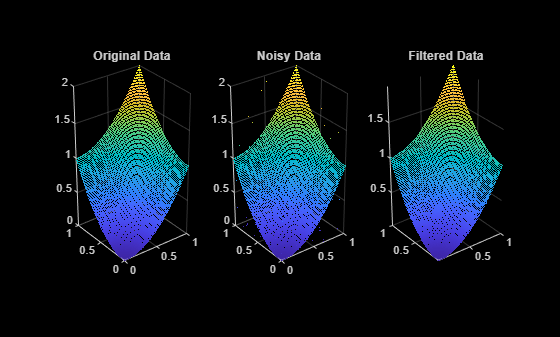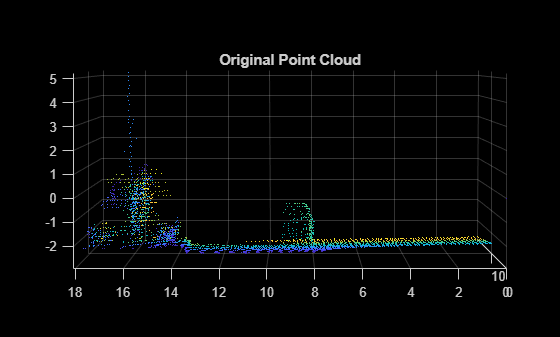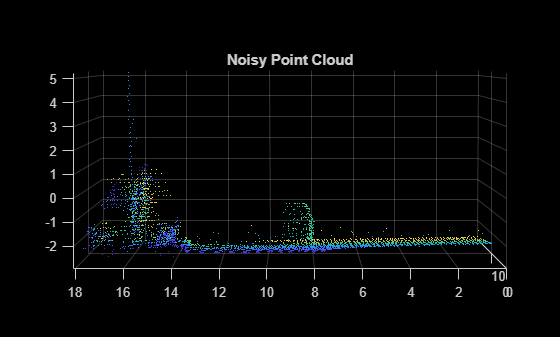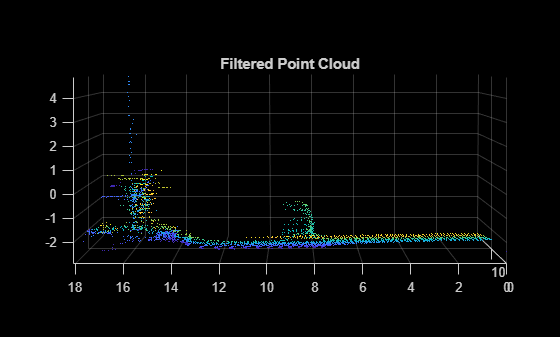# pcmedian

Median filtering 3-D point cloud data

## Syntax

``ptCloudOut = pcmedian(ptCloudIn)``
``ptCloudOut = pcmedian(___,Name,Value)``

## Description

example

````ptCloudOut = pcmedian(ptCloudIn)` performs median filtering of 3-D point cloud data. The function filters each channel of the point cloud individually. The output is a filtered point cloud. Each output location property value is the median of neighborhood around the corresponding input location property value. The `pcmedian` function doesn't pad zeros on the edges. Rather, it operates only on the available neighborhood values.If the input point cloud is an organized point cloud, the `pcmedian` function uses N-by-N neighborhood method. If the point cloud is unorganized, the function uses radial neighborhood method.```

example

````ptCloudOut = pcmedian(___,Name,Value)` specifies options using one or more name-value pair arguments. For example, `'FilterSize',3` sets the size of the median filter for organized point clouds to 3.```

## Examples

collapse all

Use the median filter to remove noise from a point cloud. First, add random noise to a point cloud. Then, use the `pcmedian` function to filter the noise.

Create a point cloud.

```gv = 0:0.01:1; [X,Y] = meshgrid(gv,gv); Z = X.^2 + Y.^2; ptCloud = pointCloud(cat(3,X,Y,Z));```

Add random noise along the z-axis.

```temp = ptCloud.Location; count = numel(temp(:,:,3)); temp((2*count) + randperm(count,100)) = rand(1,100); temp(count + randperm(count,100)) = rand(1,100); temp(randperm(count,100)) = rand(1,100); ptCloudA = pointCloud(temp);```

Apply the median filter and display the three point clouds (original, noisy, and filtered).

```ptCloudB = pcmedian(ptCloudA); subplot(1,3,1) pcshow(ptCloud) title('Original Data') subplot(1,3,2) pcshow(ptCloudA) title('Noisy Data') subplot(1,3,3) pcshow(ptCloudB) title('Filtered Data')```Load point cloud data into the workspace.

```ptCloud = pcread('highwayScene.pcd'); roi = [0 20 0 20 -5 15]; indices = findPointsInROI(ptCloud,roi); ptCloud = select(ptCloud,indices); ptCloud = pcdownsample(ptCloud,'gridAverage',0.2);```

Display the point cloud data. Each point is color-coded based on its x-coordinate.

```figure pcshow(ptCloud.Location,ptCloud.Location(:,1)) view(-90,2) title('Original Point Cloud')```Add noise along z-channel in the interval (`a`,`b`). Values of `a` and `b` are chosen to make the noise appear close to the ground.

```temp = ptCloud.Location; count = numel(temp(:,3)); a = -2.5; b = -2; temp((2*count)+randperm(count,200)) = a+(b-a).*rand(1,200); ptCloudA = pointCloud(temp);```

Display the noisy point cloud. Each point is color-coded based on its x-coordinate.

```figure pcshow(ptCloudA.Location,ptCloudA.Location(:,1)) view(-90,2) title('Noisy Point Cloud')```Apply median filter on the point cloud.

`ptCloudB = pcmedian(ptCloudA,'Dimensions',3,'Radius',1);`

Display the filtered point cloud. Each point is color-coded based on its x-coordinate.

```figure pcshow(ptCloudB.Location, ptCloudB.Location(:,1)) view(-90,2) title('Filtered Point Cloud')```## Input Arguments

collapse all

Point cloud, specified as a `pointCloud` object with at least one valid point. If the input point cloud is organized, the size of the point cloud must be at least 3-by-3-by-3.

### Name-Value Arguments

Specify optional pairs of arguments as `Name1=Value1,...,NameN=ValueN`, where `Name` is the argument name and `Value` is the corresponding value. Name-value arguments must appear after other arguments, but the order of the pairs does not matter.

Before R2021a, use commas to separate each name and value, and enclose `Name` in quotes.

Example: `'FilterSize'`,`3` specifies a median filter size of 3.

Point cloud dimensions of interest, specified as a vector of integers in the range [1 3]. The values 1, 2, and 3 correspond to the x-, y-, and z-axis respectively. You must specify dimensions in ascending order.

Data Types: `single` | `double` | `int8` | `int16` | `int32` | `int64` | `uint8` | `uint16` | `uint32` | `uint64`

Size of the median filter for an organized point cloud, specified as an odd integer in the range [3, N]. N is the smallest of channel dimensions in the point cloud.

Data Types: `single` | `double` | `int8` | `int16` | `int32` | `int64` | `uint8` | `uint16` | `uint32` | `uint64`

Radius of the neighborhood for unorganized point cloud, specified as a positive scalar. The computation time increases when there are a lot of points inside the specified radius. So, large radius values for dense point clouds can cause high computation time and impact performance.

Data Types: `single` | `double`

## Output Arguments

collapse all

Filtered point cloud, returned as a `pointCloud` object.

## Version History

Introduced in R2020b